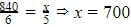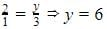Test: Direct And Inverse Proportions- 2

# Test: Direct And Inverse Proportions- 2

Test Description

## 20 Questions MCQ Test Mathematics (Maths) Class 8 | Test: Direct And Inverse Proportions- 2

Test: Direct And Inverse Proportions- 2 for Class 8 2022 is part of Mathematics (Maths) Class 8 preparation. The Test: Direct And Inverse Proportions- 2 questions and answers have been prepared according to the Class 8 exam syllabus.The Test: Direct And Inverse Proportions- 2 MCQs are made for Class 8 2022 Exam. Find important definitions, questions, notes, meanings, examples, exercises, MCQs and online tests for Test: Direct And Inverse Proportions- 2 below.
Solutions of Test: Direct And Inverse Proportions- 2 questions in English are available as part of our Mathematics (Maths) Class 8 for Class 8 & Test: Direct And Inverse Proportions- 2 solutions in Hindi for Mathematics (Maths) Class 8 course. Download more important topics, notes, lectures and mock test series for Class 8 Exam by signing up for free. Attempt Test: Direct And Inverse Proportions- 2 | 20 questions in 20 minutes | Mock test for Class 8 preparation | Free important questions MCQ to study Mathematics (Maths) Class 8 for Class 8 Exam | Download free PDF with solutions
 1 Crore+ students have signed up on EduRev. Have you?
Test: Direct And Inverse Proportions- 2 - Question 1

### When one quantity is increased, the other quantity is also increased. This proportion is called .....................

Detailed Solution for Test: Direct And Inverse Proportions- 2 - Question 1 It is called Direct proportion as it's one quantity is increased other also increases.
Test: Direct And Inverse Proportions- 2 - Question 2

### If y = x, then both are

Detailed Solution for Test: Direct And Inverse Proportions- 2 - Question 2

Since y=x, if y increases , x also increases and if y decreases x also decreases. So they are directly related.

Test: Direct And Inverse Proportions- 2 - Question 3

### 72 books are packed in 4 cartons of the same size. How many cartons are required for 360 books?

Detailed Solution for Test: Direct And Inverse Proportions- 2 - Question 3 Correct answer is 'C' because in each cartoon there are 18 books. If we divide 360 /18 than the answer is 20.
Test: Direct And Inverse Proportions- 2 - Question 4

36 men can complete a piece of work in 18 days. In how many days will 27 men complete the same work ?

Detailed Solution for Test: Direct And Inverse Proportions- 2 - Question 4 36 men can complete a piece of work in 18 days. 1 Man can complete a piece of work in 36*18 27 men can complete a piece of work =36*18/27 =36*2/3 =12*2=24
Test: Direct And Inverse Proportions- 2 - Question 5

A garrison of 500 persons had provisions for 27 days. After 3 days a reinforcement of 300 persons arrived. For how many more days will the remaining food last now?

Detailed Solution for Test: Direct And Inverse Proportions- 2 - Question 5

Let the remaining food last for x days
500 men had provision for = (27 - 3) = 24 days
(500 + 300) men had provision for x days
More men, Less days (Indirect proportion)
∴ 800 : 500 :: 24 : x
⇔ (800 × x) = (500 × 24)
⇔ x = (500 × 24)/800
⇔ x = 15

Test: Direct And Inverse Proportions- 2 - Question 6

12 men can dig 8 meters long trench in a day. How many men should be employed for digging 50 meter long trench of the same type in one day?

Test: Direct And Inverse Proportions- 2 - Question 7

In an army camp, there are 320 soldiers. They have sufficient food for 80 days. If after 20 days, 20 soldiers left the camp, for how many more days will the food last?

Detailed Solution for Test: Direct And Inverse Proportions- 2 - Question 7

Food for 320 students= 80 days

20 students left the campus

Number of students = 320 - 20 = 300 students

Food will last for (80 - 20) = 60 days fpr 320 students

Food will last for X days for 300 students

⇒ X = (320 x 60)/300 = 64

Food for 300 students= 64 days

Test: Direct And Inverse Proportions- 2 - Question 8

A journey by bus takes 45 minutes at 40 km/hour. How fast must a car go to undertake the same journey in 25 minutes?

Test: Direct And Inverse Proportions- 2 - Question 9

The cost of 5 metres of a particular quality of cloth is Rs 210. Find the cost of 2 metres of cloth of the same type.

Test: Direct And Inverse Proportions- 2 - Question 10

A mixture of paint is prepared by mixing 1 part of red pigments with 8 parts of base. How many parts of base will be used in mixture by mixing 4 part of red pigment?

Test: Direct And Inverse Proportions- 2 - Question 11

6 pipes are required to fill a tank in 1 hour 20 minutes. How long will it take if only 5 pipes of the same type are used?

Test: Direct And Inverse Proportions- 2 - Question 12

Two quantities x and y are said to be in ___________ if they increase (decrease) together in such a manner that the ratio of their corresponding values remains constant.

Test: Direct And Inverse Proportions- 2 - Question 13

Two quantities x and y are said to be in _________ if an increase in x causes a proportional decrease in y (and vice-versa) in such a manner that the product of their corresponding values remains constant.

Test: Direct And Inverse Proportions- 2 - Question 14

An electric pole, 14 metres high, casts a shadow of 10 metres. Find the height of a tree that casts a shadow of 15 metres under similar conditions.

Test: Direct And Inverse Proportions- 2 - Question 15

A machine in a soft drink factory fills 840 bottles in six hours. How many bottles will it fill in five hours?

Detailed Solution for Test: Direct And Inverse Proportions- 2 - Question 15

It’s direct proportionBottles

Test: Direct And Inverse Proportions- 2 - Question 16

Suppose 2 kg of sugar contains 9 × 106 crystals. How many sugar crystals are there in 1.2 kg of sugar?

Test: Direct And Inverse Proportions- 2 - Question 17

Two persons could fit new windows in a house in 3 days. One of the persons fell ill before the work started. How long would the job take now?

Detailed Solution for Test: Direct And Inverse Proportions- 2 - Question 17

It is an inverse relationdays

Test: Direct And Inverse Proportions- 2 - Question 18

The cost of 5 metres of a particular quality of cloth is Rs 210. Find the cost of 4 metres of cloth of the same type.

Detailed Solution for Test: Direct And Inverse Proportions- 2 - Question 18

Given, price of 5m cloth = 210
price of 1m cloth = 210 / 5 = 42
price of 4m cloth = 4×42
= RS.168

Test: Direct And Inverse Proportions- 2 - Question 19

A mixture of paint is prepared by mixing 1 part of red pigments with 8 parts of base. How many parts of base will be used in mixture by mixing 7 part of red pigment?

Detailed Solution for Test: Direct And Inverse Proportions- 2 - Question 19

It is direct proportion,
So, 1/8 = 7/y
y = 8 * 7
y = 56 parts of base.

Test: Direct And Inverse Proportions- 2 - Question 20

There are 100 students in a hostel. Food provision for them is for 20 days. How long will these provisions last, if 25 more students join the group?

Detailed Solution for Test: Direct And Inverse Proportions- 2 - Question 20

Number of person = 100, Number of days = 20, = 100 * 20
Number of person = 125  ( 25 student join), let Number of days = x = 125 * X
So, 100 * 20 = 125 * X
or 2000 = 125x
or, 125x =  2000

∴ X = 2000/125 = 16
Therefore, that the last provisions will 16 dyas.

## Mathematics (Maths) Class 8

187 videos|323 docs|48 tests
 Use Code STAYHOME200 and get INR 200 additional OFF Use Coupon Code
Information about Test: Direct And Inverse Proportions- 2 Page
In this test you can find the Exam questions for Test: Direct And Inverse Proportions- 2 solved & explained in the simplest way possible. Besides giving Questions and answers for Test: Direct And Inverse Proportions- 2, EduRev gives you an ample number of Online tests for practice

## Mathematics (Maths) Class 8

187 videos|323 docs|48 tests